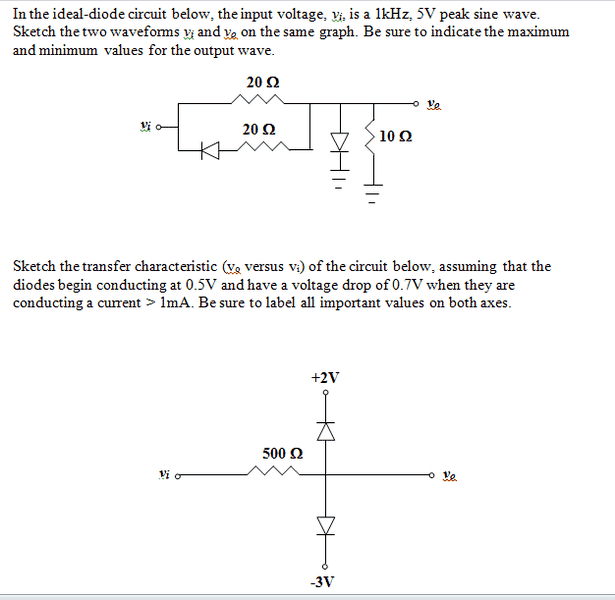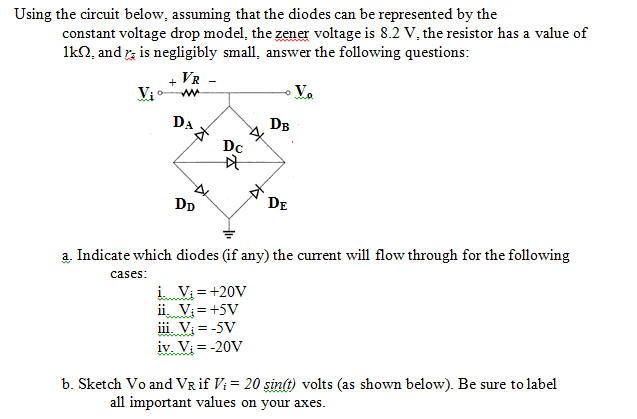# Sample exam problems (diodes)

• asdf12312

## Homework Statement## The Attempt at a Solution

My instructor gave us some sample problems he said might be on the exam, I tried working them out but I was having difficulty with 3 of them:

For the 1st problem, when there is positive value for voltage, I got the 1st diode as off (open circuit) and the 2nd diode as on (short circuit). This would mean Vout is always 0 for positive voltage. When the voltage becomes negative, the 1st diode becomes a short and the 2nd becomes open circuit. So 20||20=10 and Vo=(0.5)Vin using voltage division. Graph for Vout would be 0 when the 5V peak sine wave is positive curve, and a curve of half the amplitude when it is a negative curve (min=-2.5).

For the 2nd problem, I was used to working with 1K ohm resistors so the 500 ohm kinda confused me. But if the value of resistor doesn't matter, I find that diode starts conducting when Vi=2.5 and conducts fully when Vi=2.7 (+2V). This would mean graph is a linear line (Vo=Vi) until Vi=2.7 when it becomes a horizontal line. For the -3V, diode starts conducting when Vi=-2.5 and conducts fully when Vi=-2.3. Graph would look similar to the +2V one.

For the 3rd one, I had a zener diode for another problem so I am assuming there is positive value at the negative end. Also VZ=VZ0 because rz is very small. For +20V, I got current flowing through diodes B, C, D. the voltage is 20-2Vd-Vz=10.4V. For +5V, I got a negative voltage after the voltage drop across zener diode so I found no current would flow through the loop. For -5V, I got a positive voltage after the voltage gain in zener diode, so zero current again. For the last one (-20V), I found current flowing through doides A, C, E if I assume zener diodes can conduct voltage in both ways. Then the voltage is -20+Vz+2Vd=-10.4V.

(a) is A-OK.

For (b), the resistance certainly does matter.
When Vin = 0 what is the current flowing thru the lower diode? You have to try both Vd = 0.5V and Vd = 0.7V and pick the right one.

Then, run Vi from -5V to +5V to get the output. What is Vout when Vin = -1.9V? When Vin = -2.1V?

Does the top diode ever turn on?

You gt (c) right also.

Why would the zener not conduct current the same way for a negative 20V input as for a positive 20V input? The zener current is in the same direction for both +20 and - 20V inputs.

(b) I don't really understand this type of circuit, usually with the previous problems i had only 1 diode and voltage battery. When vi=0, the current through lower diode would be (0-Vd+3) diovided by resistance. I'm not really sure what you mean by choosing Vd=0.5 or 0.7 though. I get I=5 or 4.6mA depending on this.

When Vi=-1.9 and -2.1, current has to flow through bottom diode only. I think vout would be -2.3V for both because that's when the diode finishes conducting.

am I doing this right so far? Maybe the vout graph has to include both values then, for both the +2 and -3V sources. So I should graph both for vi=-5 to +5V. But I think my only problem is what vout would be at Vin=0V, -2.3V or just 0V.

also, for (c), would I be right in thinking that if I graphed VR, it would look like the sin wave input except with amplitude of 10.4V? And V0 would be a constant value instead of a curve, +/- 9.6V depending on the sine value.

Last edited:
If the input is -1.9V, and the voltage drop across the bottom diode is 0.5V, what would be the current?

And what about the top diode? What input voltage would turn it on?

(b) I don't really understand this type of circuit, usually with the previous problems i had only 1 diode and voltage battery. When vi=0, the current through lower diode would be (0-Vd+3) diovided by resistance. I'm not really sure what you mean by choosing Vd=0.5 or 0.7 though. I get I=5 or 4.6mA depending on this.

Remember what the problem stated: if i < 1 mA, Vd = 0.5 and if i > 1 mA then Vd = 0.7v. So which do you think you should choose?

I see now. If input is -1.9V, the current through the bottom diode is (-1.9-Vd+3)/500=1.2mA. But this is >1mA..so I should choose the bottom diode Vd=0.7V right? For the top diode using a similar problem I was assigned I found it starts conducting at 2.5V.

For the top diode: what's the highest voltage the anode can assume?